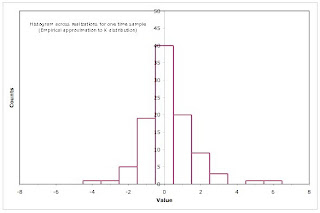## Saturday, May 08, 2010

### A Nonergodic, Stationary Random Process

1.0 Introduction
Joan Robinson famously distinguished between economic models set in logical and historical time. According to Robinson, the distinguishing feature of Keynes’ General Theory is its setting in historical time. Building on Paul Davidson, one might say that one sign that a model is set in historical time is that it generates nonergodic stochastic processes.

This post explains that claim somewhat by presenting a simple example of a stationary nonergodic stochastic processes, namely a Spherically Invariant Random Process (SIRP).

2.0 Spherically Invariant Random Processes (SIRPs)
A stochastic process, {X(i), i = 0, 1, ..., n - 1}, is an indexed set of random variables. Typically, the index is taken to be time. Each random variable X(i) has an associated probability distribution, which can be specified by a Cumulative Distribution Function (CDF):
Fi(x) = Prob( X(i) ≤ x),
where Fi is the CDF. The derivative of the CDF is the Probability Density Function (PDF). (I guess differentiation, in this sense, is the inverse operation of Lebesque-Stieltjes integration.)

If the stochastic process {X(i), i = 0, 1, ..., n - 1} is a SIRP, it can be represented as the product
X(i) = Y Z(i), i = 0, 1, ..., n - 1,
where Y is a random variable not indexed on time and Z(i) is from a Gaussian distribution with a mean of zero.

2.1 A Single Realization
To consider an example, I picked a distribution for Y, namely the Chi distribution. (A random variable is from a Chi distribution if it is the square root of a random variable from a Chi Squared distribution.) Arbitrarily, I set the degrees of freedom of the corresponding Chi Squared distribution to be 2. For simplicity, let the variance of the normally distributed random variables {Z(i), i = 0, 1, ..., n - 1} be unity.

Figure 1 shows 100 time samples generated from this stochastic process. A single realization y of the Chi distribution is generated for each realization of the SIRP. The time samples consist of the product of this value and 100 realizations generated from a standard normal distribution. Figure 2 is a histogram formed from these 100 time samples. Does the histogram look bell-shaped?Figure 1: A Realization of a Random ProcessFigure 2: Distribution Over Time

2.2 Many Realizations
I generated 100 realizations of this SIRP, each consisting of 100 time samples. Consider a fixed time sample, say i = 4. The 100 realizations of the SIRP allow one to create a sample of the value of the SIRP at this time sample. Figure 3 shows the resulting distribution. The distribution shown reflects variation resulting from the Chi distribution, as well as the variation in the Gaussian distribution. I don’t find it obvious to the eye that this distribution is peaked differently (has a different kurtosis) than a Gaussian distribution.Figure 3: Distribution Across Realizations

2.3 Nonergodic Stochastic Processes
Figures 2 and 3 are constructed from two random samples, each of 100 points. These samples can each be used to estimate parameters of the stochastic process – for example, the CDF at specified values. If the stochastic process were ergodic such estimates would converge as the sample sizes increased. That is, an estimator based on a large enough number of time samples from a single realization would be equally as good, in some sense, as an estimator based on data across a large enough number of realization at a specified time sample.

But this SIRP is nonergodic. Figure 4 shows the CDFs estimated from the two random samples. The Kolmogorov-Smirnov statistic provides a formal statistical test for deciding whether the difference between these two estimates of the CDF can be explained by random variation. And that test rejects the null hypothesis at a 5% level of statistical significance. To summarize – this post has presented a Monte Carlo demonstration that a SIRP can be nonergodic. The question raised for the economist is whether their theories apply if stochastic processes observed in actual economies (for example, the prices of stocks) are nonergodic.Figure 4: Empirical Cumulative Distribution Functions (CDF)

References
• Paul Davidson, "Rational Expectations: A Fallacious Foundation for Studying Crucial Decision-Making Processes", Journal of Post Keynesian Economics, V. V, N. 2 (Winter 1982-83): 182-198.
• Joan Robinson, "History versus Equilibrium", in Contributions to Modern Economics, Blackwell (1978)Anonymous said...

What is puzzling is that economists still think to explain human behaviour by using randomPablo said...

That was really helpful. I have been trying to picture a stationary non-ergodic process, so I could understand correctly the difference between those two concepts, and this made my day.

Robert Vienneau said...

Thanks for the comment. I have recently stumbled on something called a Doubly Stochastic Poisson Process, which reminds me of a SIRP. Cosma Shalizi has a post referencing mine.